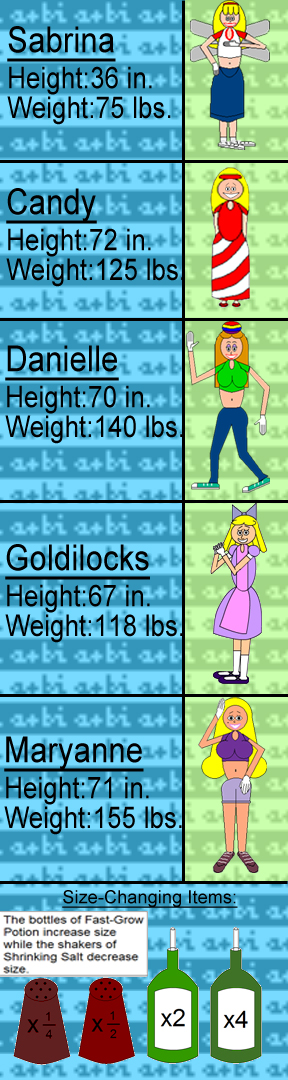# Mass Multiplication or Wacky Weight-Changing Women

#### Difficulty: MediumThese are the heights & weights of 5 chosen characters in this cartoon series. But what if they changed size? How tall or heavy would they be if they magically grew by drinking fast-grow potion? How small or light would they be if they sprinkled themselves with shrinking salt? The numbers on the items multiply their sizes.

As they change size, their mass, volume, or weight is determined by this math formula: M=k × L^d

M=Mass

k=Original Size

L=Size Multiplier

d=Number of Dimensions

An object or character's weight and volume are always multiplied by the same number as the mass.

The characters are 3-dimensional, therefore, in this case, d=3.

When measuring height, d=1, since height is 1-dimensional.

To keep things simplified, I only used 1 unit for height(inches), and 1 unit for weight(Earth pounds).

Your goal is to calculate how much these characters would weigh if they doubled, quadrupled, halved, or quartered their normal sizes; and also, how tall would they be?